## 1. Overview

Mathematics is everywhere in the Machine Learning field: input and output data are mathematical entities, as well as the algorithms to learn and predict.

But what happens when we need to deal with linguistic entities such as words? How can we model them as mathematical representations? The answer is we convert them to vectors!

In this tutorial, we’ll learn the main techniques to represent words as vectors and the pros and cons of each method, so we know when to apply them in real life.

## 2. One-Hot Vectors

### 2.1. Description

This technique consists of having a vector where each column corresponds to a word in the vocabulary.

Let’s see an example with these sentences:

(1) John likes to watch movies. Mary likes movies too.
(2) Mary also likes to watch football games.

This would be our vocabulary:So we will have this vector, where each column corresponds to a word in the vocabulary: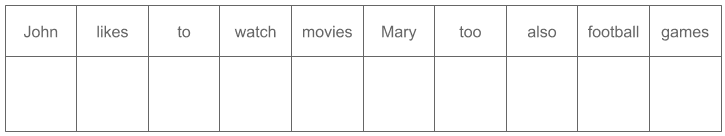Each word will have a value of 1 for its own column and a value of 0 for all the other columns.

The vector for the word “likes” would be: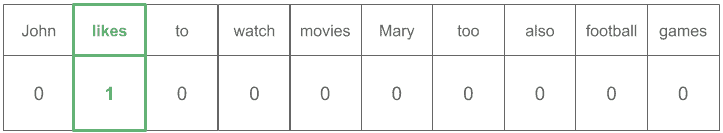Whereas the vector for “watch” would be: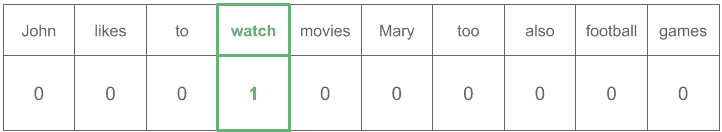There are different ways to create vocabularies, depending on the nature of the problem. This is an important decision to make because it’ll affect the performance and speed of our system.

In the next sections, we’ll review different methods to create that vocabulary.

### 2.2. Stop Words Removal

Stop words are those we ignore because they are not relevant to our problem. Typically we’ll remove the most common ones in the language: “the”, “in”, “a” and so on.

This helps to obtain a smaller vocabulary and represent words with fewer dimensions.

### 2.3. Reducing Inflectional Forms

A popular and useful technique is reducing the explosion of inflectional forms (verbs in different tenses, gender inflections, etc.) to a common word preserving the essential meaning. The main benefits of using this technique are:

• Use a shorter vocabulary, which makes the system faster
• The common word would be more present in the dataset, so we would have more examples during training

There are two main specific techniques to achieve this: Stemming and Lemmatization.

Stemming is the process of truncating words to a common root named “stem”.

• All words will share the same root as a stem, but the stem could be a word out of the language
• It’s easy to calculate but has the drawback of producing non-real words as stems

Let’s see a stemming example in which the stem is still a real word in the language (“Connect”):Now we’ll see a case with a stem out not being a word (“Amus”):Lemmatization consists of reducing words to their canonical, dictionary, or citation form named “lemma”.

• Words could or could not share the same root as the lemma, but the lemma is a real word
• Typically this is a more complicated process, as it requires big databases or dictionaries to find lemmas

See next a case of lemmatization in which words with inflection share the root with the lemma (“Play”):Finally, we’ll see an example of a lemma (“Go”) not sharing the root with the words with inflection:### 2.4. Lowercase Words

Lowercasing the vocabulary is usually a good way to significantly reduce it without meaning loss.

However, if uppercased words are relevant to the problem, we shouldn’t use this optimization.

### 2.5. Strategies to Handle Unknown Words

The most common strategies to deal with Out Of Vocabulary (OOV) words are:

• Raise an error: This would imply to stop the process, create a column for that word, and start again
• Ignore the word: Treat it as if it was a stop word
• Create a special word for unknown values: We’d create the word “UNK” (“Unknown”), and every OOV word would be considered that word

### 2.6. Pros and Cons

The drawbacks are:

• Extremely large and sparse vectors: We could easily be working with 100M dimensional vectors per word
• Lack of geometrical properties: Similar words don’t have closer vectors in space

• Straightforward approach
• Fast to compute
• Easy to calculate over a cluster in a distributed way
• Highly interpretable

## 3. Word Embeddings

### 3.1. Description

Word embeddings are just vectors of real numbers representing words.

They usually capture word context, semantic similarity, and relationship with other words.

### 3.2. Embeddings Arithmetic

One of the coolest things about word embeddings is that we can apply semantic arithmetic to them.

Let’s have a look at the relationships between word embedding vectors: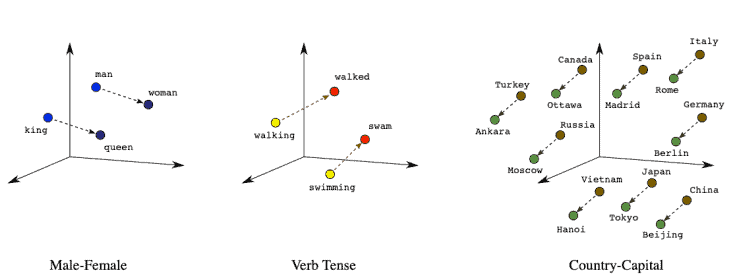We can notice in the Male-Female graph, the distance between “king” and “man” is very similar to the distance between “queen” and “woman”. That difference would correspond to the concept of “royalty”: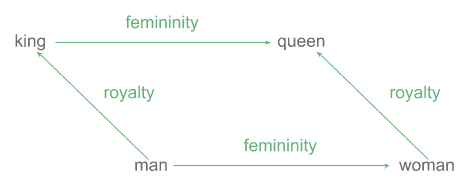We could even approximate the vector for “king” applying these operations:This ability to express arithmetically and geometrically semantic concepts is what makes word embeddings especially useful in Machine Learning.

### 3.3. Pros and Cons

Word embeddings drawbacks are:

• They require a lot of training data compared to one-hot vectors
• They are slower to compute
• Low interpretability

• Preservation of semantic and syntactic relationships
• We can measure how close are two words in terms of meaning
• We can apply vector arithmetic

## 4. Word2vec

Let’s now learn one of the most popular techniques to create word embeddings: Word2vec.

The key concept to understand how it works is the distributional hypothesis in linguistics: words that are used and occur in the same contexts tend to purport similar meanings“.

There are two methods in Word2vec to find a vector representing a word:

• Continuous Bag Of Words (CBOW): the model predicts the current word from the surrounding context words
• Continuous skip-gram: the model uses the current word to predict the surrounding context words

The architecture is a very simple shallow neural network with three layers: input (one-hot vector), hidden (with N units of our choice), and output (one-hot vector).

The training will minimize the difference between the expected output vectors and the predicted ones, leaving as a side-product the embeddings in a weights matrix (in red):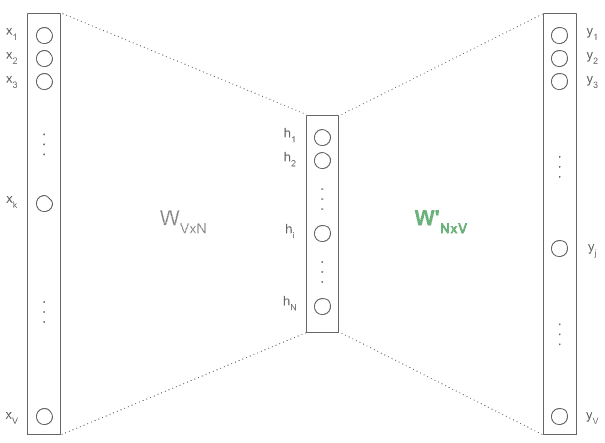So we’ll have two weight matrices: Matrixbetween the input and hidden layer, and Matrixbetween the hidden and output layer.

After training, the matrixwith sizewill have one column per word, which are the embeddings for each word.

### 4.1. CBOW Architecture:

The whole concept of CBOW is that we know the context of a word (the surrounding words), and our goal is to predict that word.

For example, imagine we train with the former text:

John likes to watch movies. Mary likes movies too.and we decide to use a window of 3 (meaning we’ll pick one word before the target word and one word after it).

These are the training examples we’d use:

John ____ to     (Expected word: "likes")

likes ____ watch (Expected word: "to")

to ____ movies   (Expected word: "watch")

...

all encoded as one-hot vectors: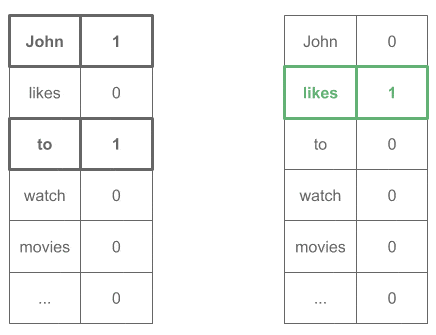Then we would train this network as any other neural classifier, and we would obtain our word embeddings in the weights matrix.

### 4.2. Skip-Gram Architecture:

The only difference in this approach is that we’d use the word as input and the context (surrounding words) as output (predictions).

Therefore, we’d generate these examples:

"likes" (Expected context: ["John", "to"])
"to"    (Expected context: ["likes", "watch"])
"watch" (Expected context: ["to", "movies"])
...

Which expressed as one-hot vectors would look like: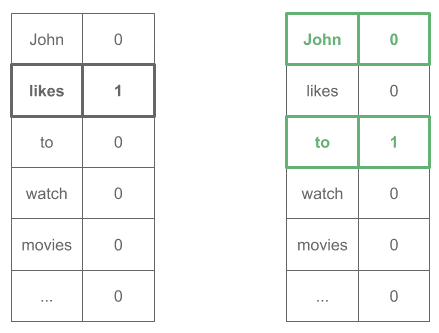• Sub-sampling: Very frequent words may be subsampled to increase training speed
• Dimensionality: Quality of word embedding increases with hidden dimensions, but it will diminish after some point. Typical values of hidden dimensions are in the range: 100-1,000, being very popular embeddings of size 100, 200, and 300
• Context window: According to the authors’ note, the recommended value is 10 for skip-gram and 5 for CBOW
• Custom domains: Despite having lots of pre-trained embeddings available for free, we could decide to train a custom one for a specific domain, so it better captures the nature of our text semantics and syntactic relationships, improving our system’s performance

## 5. Other Word Embedding Methods

### 5.1. GloVe

GloVe embeddings relate to the probabilities that two words appear together. Or simply put: embeddings are similar when their words appear together often.

Pros:

• Training time is shorter than Word2vec
• Some benchmarks are showing it does better in semantic relatedness tasks

Cons:

• It has a larger memory footprint need, which could be an issue when using large corpora

### 5.2. FastText

fastText is an extension of Word2vec consisting of using n-grams of characters instead of whole words.

Pros:

• Enables training of embeddings on smaller datasets
• Helps capture the meaning of shorter words and allows to understand suffixes and prefixes
• Good generalization to unknown words

Cons:

• It is slower than Word2vec, as every word produces many n-grams
• Memory requirements are bigger
• The choice of “n” is key

### 5.3. ELMo

ELMo solves the problem of having a word with different meanings depending on the sentence.

Consider the semantic difference of the word “cell” in: “He went to the prison cell with his phone” and “He went to extract blood cell samples”.

Pros:

• It takes into account word order
• Word meaning is more accurately represented

Cons:

• We need the model we used to train the vectors also after training since the models generate vectors for a word based on context

### 5.4. Other Techniques

There are a few additional techniques that aren’t as popular:

## 6. Conclusion

In this tutorial, we learned the two main approaches to generate vectors from words: one-hot vectors and word embeddings, as well as the main techniques to implement them.

We also considered the pros and cons of those techniques, so now we are ready to apply them wisely in real life.